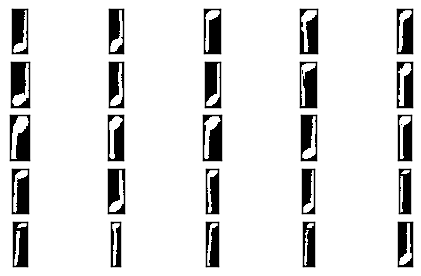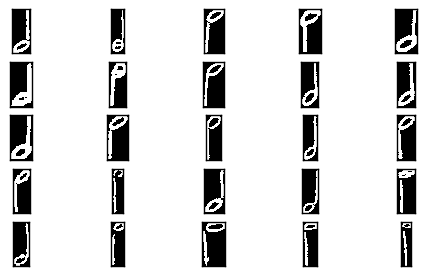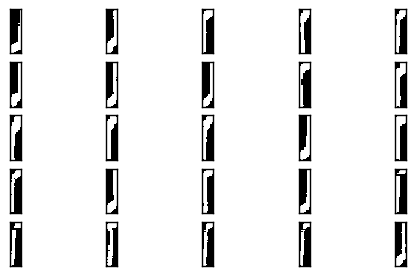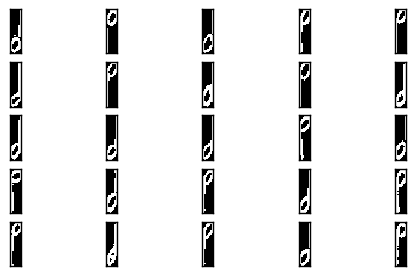# MUSCIMA++ Tutorial¶

This is a tutorial for using the `muscima` package to work with the MUSCIMA++ dataset.

```import os
from muscima.io import parse_cropobject_list

# Change this to reflect wherever your MUSCIMA++ data lives
CROPOBJECT_DIR = os.path.join(os.environ['HOME'], 'data/MUSCIMA++/v0.9/data/cropobjects')

cropobject_fnames = [os.path.join(CROPOBJECT_DIR, f) for f in os.listdir(CROPOBJECT_DIR)]
docs = [parse_cropobject_list(f) for f in cropobject_fnames]
```

Let’s do something straightforward: symbol classification.

## Symbol Classification¶

Let’s try to tell apart quarter notes from half notes.

However, notes are recorded as individual primitives in MUSCIMA++, so we need to extract notehead-stem pairs from the data using their relationships. Quarter notes are all `full-notehead`-`stem` pairs with no beam or flag. Half-notes are all `empty-notehead`-`stem` pairs.

After we extract the note classes, we will need to compute features for classification. To do that, we first need to “draw” the symbols in the appropriate relative positions. Then, we can extract whatever features we need.

Finally, we train a classifier and evaluate it.

### Extracting notes¶

```# Bear in mind that the outlinks are integers, only valid within the same document.
# Therefore, we define a function per-document, not per-dataset.

def extract_notes_from_doc(cropobjects):
"""Finds all ``(full-notehead, stem)`` pairs that form
quarter or half notes. Returns two lists of CropObject tuples:
one for quarter notes, one of half notes.

:returns: quarter_notes, half_notes
"""
_cropobj_dict = {c.objid: c for c in cropobjects}

notes = []
for c in cropobjects:
_has_stem = False
_has_beam_or_flag = False
stem_obj = None
_o_obj = _cropobj_dict[o]
if _o_obj.clsname == 'stem':
_has_stem = True
stem_obj = _o_obj
elif _o_obj.clsname == 'beam':
_has_beam_or_flag = True
elif _o_obj.clsname.endswith('flag'):
_has_beam_or_flag = True
if _has_stem and (not _has_beam_or_flag):
# We also need to check against quarter-note chords.
# for multiple inlinks will do the trick.
notes.append((c, stem_obj))

quarter_notes = [(n, s) for n, s in notes if n.clsname == 'notehead-full']
half_notes = [(n, s) for n, s in notes if n.clsname == 'notehead-empty']
return quarter_notes, half_notes

qns_and_hns = [extract_notes_from_doc(cropobjects) for cropobjects in docs]
```

Now, we don’t need the `objid` anymore, so we can lump the notes from all 140 documents together.

```import itertools
qns = list(itertools.chain(*[qn for qn, hn in qns_and_hns]))
hns = list(itertools.chain(*[hn for qn, hn in qns_and_hns]))

len(qns), len(hns)
```
```(4320, 1181)
```

It seems that we have some 4320 isolated quarter notes and 1181 isolated half-notes in our data. Let’s create their images now.

### Creating note images¶

Each notehead and stem CropObject has its own mask and its bounding box coordinates. We need to combine these two things, in order to create a binary image of the note.

```import numpy

def get_image(cropobjects, margin=1):
"""Paste the cropobjects' mask onto a shared canvas.
There will be a given margin of background on the edges."""

# Get the bounding box into which all the objects fit
top = min([c.top for c in cropobjects])
left = min([c.left for c in cropobjects])
bottom = max([c.bottom for c in cropobjects])
right = max([c.right for c in cropobjects])

# Create the canvas onto which the masks will be pasted
height = bottom - top + 2 * margin
width = right - left + 2 * margin
canvas = numpy.zeros((height, width), dtype='uint8')

for c in cropobjects:
# Get coordinates of upper left corner of the CropObject
# relative to the canvas
_pt = c.top - top + margin
_pl = c.left - left + margin
# We have to add the mask, so as not to overwrite
# previous nonzeros when symbol bounding boxes overlap.

canvas[canvas > 0] = 1
return canvas

qn_images = [get_image(qn) for qn in qns]
hn_images = [get_image(hn) for hn in hns]
```

Let’s visualize some of these notes, to check whether everything worked. (For this, we assume you have matplotlib. If not, you can skip this step.)

```import matplotlib.pyplot as plt

plt.show()

n_rows = n_masks // row_length + 1
fig = plt.figure()
plt.subplot(n_rows, n_cols, i+1)
# Let's remove the axis labels, they clutter the image.
for ax in fig.axes:
ax.set_yticklabels([])
ax.set_xticklabels([])
ax.set_yticks([])
ax.set_xticks([])
plt.show()
```
```show_masks(qn_images[:25])
```It seems that the extraction went all right.

### Feature Extraction¶

Now, we need to somehow turn the note images into classifier inputs.

Let’s get some inspiration from the setup of the HOMUS dataset. In their baseline classification experiments, the authors just resized their images to 20x20. For notes, however, this may not be such a good idea, because it will make them too short. Let’s instead resize to 40x10.

```from skimage.transform import resize

qn_resized = [resize(qn, (40, 10)) for qn in qn_images]
hn_resized = [resize(hn, (40, 10)) for hn in hn_images]

# And re-binarize, to compensate for interpolation effects
for qn in qn_resized:
qn[qn > 0] = 1
for hn in hn_resized:
hn[hn > 0] = 1
```

How do the resized notes look?

```show_masks(qn_resized[:25])
```### Classification¶

We now need to add the output labels and make a train-dev-test split out of this.

Let’s make a balanced dataset, to keep things simpler.

```# Randomly pick an equal number of quarter-notes.
n_hn = len(hn_resized)
import random
random.shuffle(qn_resized)
qn_selected = qn_resized[:n_hn]
```

Now, create the output labels and merge the data into one dataset.

```Q_LABEL = 1
H_LABEL = 0

qn_labels = [Q_LABEL for _ in qn_selected]
hn_labels = [H_LABEL for _ in hn_resized]

notes = qn_selected + hn_resized
# Flatten data
notes_flattened = [n.flatten() for n in notes]
labels = qn_labels + hn_labels
```

Let’s use the `sklearn` package for experimental setup. Normally, we would do cross-validation on data of this small size, but for the purposes of the tutorial, we will stick to just one train/test split.

```from sklearn.model_selection import train_test_split

X_train, X_test, y_train, y_test = train_test_split(
notes_flattened, labels, test_size=0.25, random_state=42,
stratify=labels)
```

What could we use to classify this data? Perhaps a k-NN classifier might work.

```from sklearn.neighbors import KNeighborsClassifier

K=5

# Trying the defaults first.
clf = KNeighborsClassifier(n_neighbors=K)
clf.fit(X_train, y_train)
```
```KNeighborsClassifier(algorithm='auto', leaf_size=30, metric='minkowski',
metric_params=None, n_jobs=1, n_neighbors=5, p=2,
weights='uniform')
```

Let’s run the classifier now and evaluate the results.

```y_test_pred = clf.predict(X_test)
```
```from sklearn.metrics import classification_report
print(classification_report(y_test, y_test_pred, target_names=['half', 'quarter']))
```
```             precision    recall  f1-score   support

half       0.98      0.87      0.92       296
quarter       0.88      0.98      0.93       295

avg / total       0.93      0.93      0.93       591
```